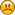###Author Topic: Can't set rotation of child corresponding to picked polygon if parent is rotated  (Read 226 times)

####Windmiller

• byte
•• Posts: 6##### Can't set rotation of child corresponding to picked polygon if parent is rotated
« on: May 20, 2020, 12:54:15 am »
Hi

I have some difficulties setting a child of a parent object when parent is rotated.

I have a custom sphere object class name TheSphere that extends Object3D and implements CollisionListener
with the following overridden functions..

Code: [Select]
`public void collision(CollisionEvent collisionEvent) {        this.mPickedCoordinate = collisionEvent.getFirstContact();    }    @Override    public boolean requiresPolygonIDs() {        return true;    }`
And from the Graphics thread I'm taking the value stored in this.mPickedCoordinate to add a Primitives.getCube() at that coordinate with the following code.

Code: [Select]
`SimpleVector touchPoint = Interact2D.reproject2D3DWS(cam, fB, (int) x, (int) y);float distance = theSphere.calcMinDistance(camPos, touchPoint, 1000);SimpleVector pickedCoordinate = theSphere.mPickedCoordinate;pickedCoordinate.sub(SimpleVector.create(theSphere.getPosX(), theSphere.getPosY(), theSphere.getPosZ()));Object3D cube = Primitives.getCube(5f);theSphere.addChild(cube);cube.translate(pickedCoordinate);world.addObject(cube);`
So when I'm touching the sphere it places a cube at the right point, but if I rotate the sphere and touch it again then a new cube will be added to the rotated side and not where I've put my finger.

How can I add a cube to the point where I've touched the sphere even when the sphere has been rotated up or down side to side doesn't matter as long as it places the cube where my finger where.

getFirstContact will always return the (not) rotated pointRegards, Morgan..
« Last Edit: May 20, 2020, 12:56:34 am by Windmiller »

####EgonOlsen

••• Posts: 12060##### Re: Can't set rotation of child corresponding to picked polygon if parent is rotated
« Reply #1 on: May 20, 2020, 07:35:29 pm »
Looks reasonable at first glance.  However, I'm not sure about the cube being a child of the sphere. This will apply the sphere's rotation to the cube as well, so assuming that you have rotated the sphere 90 degrees around Y (for example...) and are placing a cube at the position on the sphere that is now facing towards you, making the cube a child of the sphere will apply the same rotation to it, making it rotate the same 90 degrees, which will make him appear on the side in the end...or wouldn't it?

I'm not sure how to handle it. I guess (if that's the actual problem and I'm not totally off here) you could calculate the cube's translation like you do now, get the world transformation matrix of the sphere, invert it, matmul the result with the calculated point and translate the cube by that instead...maybe...

####Windmiller

• byte
•• Posts: 6##### Re: Can't set rotation of child corresponding to picked polygon if parent is rotated
« Reply #2 on: May 20, 2020, 11:42:30 pm »
Yes! Thank you Egon, the result is just fabulousI made it with the following code..

Code: [Select]
`SimpleVector touchPoint = Interact2D.reproject2D3DWS(cam, fB, (int) x, (int) y);float distance = theSphere.calcMinDistance(camPos, touchPoint, 1000);SimpleVector pickedCoordinate = theSphere.mPickedCoordinate;pickedCoordinate.matMul(theSpehere.getWorldTransformation().invert());Object3D cube = Primitives.getCube(5f);theSphere.addChild(cube);cube.translate(pickedCoordinate);world.addObject(cube);`
You saved my game, really big thanks to you.

Regards, Morgan

####EgonOlsen

••